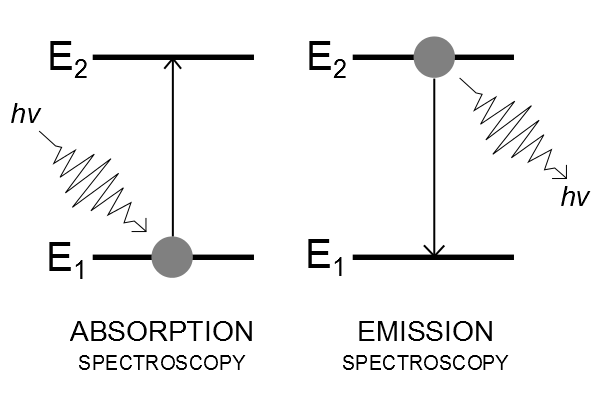# Difference Between Absorption and Emission

## Main Difference – Absorption vs Emission

Absorption and emission are two common phenomena associated with electron transitions within energy levels of an atom. Each atom is made up of a dense nucleus and a vast area of empty space which consists of energy shells where electrons reside. The energy shells closer to the nucleus are less in energy and the energy increases as further it gets from the nucleus. Consequently, the electrons residing in the lower energy levels carry lower energy and the ones occupying the higher energy levels carry a higher amount of energy respectively. Therefore, an electron in a lower energy level has to absorb energy in order to move to a higher energy level and similarly an electron in a higher energy level has to emit an equivalent amount of energy in order to move down to a lower energy level. This is the main difference between absorption and emission.

## What is Absorption

The energy of the orbitals around the nuclei of atoms are discrete. It means that this energy is not continuously variating and takes certain values. The electrons residing in these orbitals also carry the same amount of discrete energy. When electrons interact with electromagnetic radiation, they absorb its energy and are able to elevate into higher energy level orbitals within the atom. For this to happen, the energy carried by the electromagnetic wave has to be equal to the energy gap between the orbitals. It has been proven that electromagnetic waves also carry discrete amounts of energy rather than energy in a continuous form. In addition, this transfer of energy takes place between the electron and the wave at an optimum state.

Therefore, the process in which an electron accepts a discrete amount of energy (delivered to it by an electromagnetic wave) and elevates itself to a higher energy level is known as ‘absorption’. Depending on the energy provided by the electromagnetic wave, the electron can either move up to the next energy level or to a higher one skipping several levels. However, the energy provided by the electromagnetic waves need to match the transitioning energy gap between the orbitals. If enough energy is supplied by the energy source, the electrons might be able to absorb this energy and get excited to an extent where it leaves the atomic orbitals. This is called ‘ionization’.## What is Emission

The same explanation holds true for the case of emission as well. This is the inverse process of absorption, where energy is given out. Therefore, if an electron in a higher energy level needs to move downwards to an orbital with lower energy, it needs to release its additional energy. This additional energy is also been released as an electromagnetic wave which is able to carry a discrete amount of energy. Like in the case of absorption, the amount of energy released depends on how far the electron needs to fall. The deeper it needs to fall, the more energy it has to release.

However, the release of this energy does not need to occur at once. The electron could also fall by releasing energy time to time. And each time it releases energy, it would do so in the form of electromagnetic waves. Therefore, the higher emissions would be in the range of X-rays etc and the lower energy emissions would be in the range of IR rays etc. LASERS are produced through stimulated emission. What happens here is that electrons emit energy under the influence of an external light beam (electromagnetic wave), where the waves are emitted in parallel.

## Difference Between and Emission

### Definition

Absorption involves the absorbing of energy by the electrons.

Emission refers to the release of energy by the electrons.

### Direction of movement

When electrons absorb energy they move up towards a higher energy level.

When electrons emit energy they move down towards a lower energy level.

### Association with Oxidation numbers

Absorption favours the increase of oxidation number through the process of ionization.

Emission cannot be linked to an oxidation number.

### Action under Stimulation

Absorption doesn’t occur under stimulated energy.

When emission takes place under stimulation, it produces LASERS.Image Courtesy:

Image 1 by Mysterioso – Own work, (CC BY-SA 3.0) via Commons Wikimedia